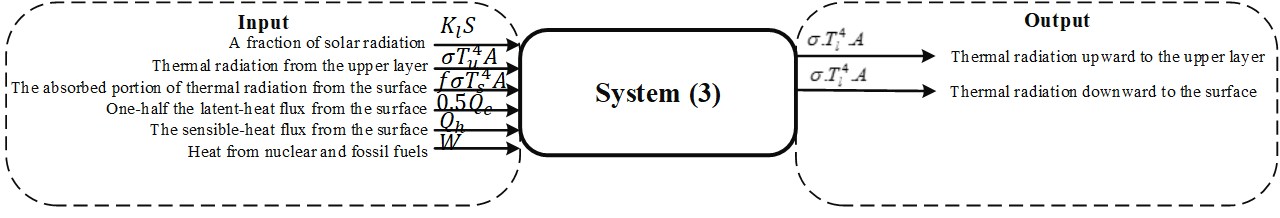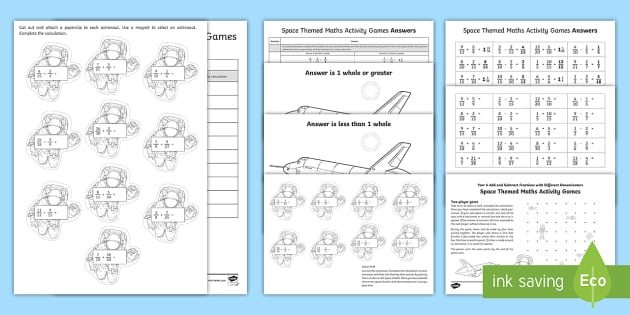# Fractions 2

Materials

• M & Ms of different colors
• Hershey Candy Bars
• Magnetic fraction bars

Activity

To add fractions there are Three Simple Steps: Step 1: Make sure the bottom numbers (the denominators) are the same, Step 2: Add the numerators, put that answer over the denominator, Step 3: Simplify the fraction (if needed). If the 2 numbers of the fraction end in 0 and/or 5, you can divide by 5 5. 14 9 70 5 45 5 70 45 Hint 4 If both numbers end in zeros, you can cancel the zeros in pairs, one from the top and one from the bottom. This is the same as dividing them by 10 10 for each cancelled pair. 25 2 50 2 4 2 50 4 Hint 5 If you have tried to.

I start by passing out a paper candy bar to everyone that has 12 parts.

## Fractions - Practice With Math Games

We talk about how a candy bar is the whole and they they cut it in half so they see that 6 pieces=1 half. I model with a real candy bar.

We talk about them splitting this with a friend so they would have EQUAL PARTS. Then I tell them 2 other friends come over so they have to split each half into equal parts again. As we do each split, I draw a picture of the fraction and label it.

Once we have divided the candy bar into twelve pieces and I have shown them how we went from 1/2 to 1/12, I use magnetic fraction bars to show them briefly how 1/12 is much smaller than 1/2.

Once they get this down and have enjoyed a real candy bar, I then take small bags of M & Ms and pass them out to pairs of kids. We divide our candy into groups by color and then figure out which fraction of the different colors everyone has. For example, if they have 24 total candies and 8 of them are red, I show them that 8/24 are red. Those that are ready always seem to tell me that this is 1/3. (I divide candy in advance so there is always an even number in bags, but not always the same number so some groups may get 24, others 30, others 36, etc.).

When we have figured out the fraction of each color (I have them draw it out on paper with crayons and label the pictures), I let them eat their fractions. My kids always love this since it involves food and they always get fractions too!

A fraction like 34 says we have 3 out of the 4 parts the whole is divided into.

### To add fractions there are Three Simple Steps:

• Step 1: Make sure the bottom numbers (the denominators) are the same
• Step 2: Add the top numbers (the numerators), put that answer over the denominator
• Step 3: Simplify the fraction (if needed)

## Example:

Step 1. The bottom numbers (the denominators) are already the same. Go straight to step 2.

Step 2. Add the top numbers and put the answer over the same denominator:

14 + 14 = 1 + 14 = 24

Step 3. Simplify the fraction:

 14 + 14 = 24 = 12

... and do you see how 24 is simpler as 12 ? (see Equivalent Fractions.)

## Example:

13 + 16## Fractions 2 - Image Results

Step 1: The bottom numbers are different. See how the slices are different sizes?

 13 + 16 = ?

We need to make them the same before we can continue, because we can't add them like that.

The number '6' is twice as big as '3', so to make the bottom numbers the same we can multiply the top and bottom of the first fraction by 2, like this:

 × 2
 × 2

Important: you multiply both top and bottom by the same amount,
to keep the value of the fraction the same

Now the fractions have the same bottom number ('6'), and our question looks like this:

 26 + 16

The bottom numbers are now the same, so we can go to step 2.

Step 2: Add the top numbers and put them over the same denominator:

26 + 16 = 2 + 16 = 36

In picture form it looks like this:

 26 + 16 = 36

Step 3: Simplify the fraction:

36 = 12

In picture form the whole answer looks like this:

 26 + 16 = 36 = 12

### With Pen and Paper

And here is how to do it with a pen and paper (press the play button):

The bottom numbers must be the same!

'Change the bottom using multiply or divide,
But the same to the top must be applied,

'And don't forget to simplify,
Before its time to say good bye'

## Example:

13 + 15

Again, the bottom numbers are different (the slices are different sizes)!

 13 + 15 = ?

But let us try dividing them into smaller sizes that will each be the same:

 515 + 315The first fraction: by multiplying the top and bottom by 5 we ended up with 515 :

 × 5
 13 = 515× 5

The second fraction: by multiplying the top and bottom by 3 we ended up with 315 :

 × 3
 × 3

The bottom numbers are now the same, so we can go ahead and add the top numbers:

 515 + 315 = 815

## More Fractions 2 Images

The result is already as simple as it can be, so that is the answer: 815

13 + 15 = 815

## Making the Denominators the Same

In the previous example how did we know to cut them into 1/15ths to make the denominators the same? We simply multiplied the two denominators together (3 × 5 = 15).

Read about the two main ways to make the denominators the same here:

• Common Denominator Method, or the

They both work, use which one you prefer!

### Example: Cupcakes

You want to make and sell cupcakes:

• A friend can supply the ingredients, if you give them 1/3 of sales
• And a market stall costs 1/4 of sales

How much is that altogether?

We need to add 1/3 and 1/4

 1 + 1 = ? 3 4 ?

First make the bottom numbers (the denominators) the same.

Multiply top and bottom of 1/3 by 4:

 1 × 4 + 1 = ? 3 × 4 4 ?

And multiply top and bottom of 1/4 by 3:

 1 × 4 + 1 × 3 = ? 3 × 4 4 × 3 ?

## Fractions Calculator - RapidTables.com

Now do the calculations:

 4 + 3 = 4+3 = 7 12 12 12 12

Answer: 712 of sales go in ingredients and market costs.